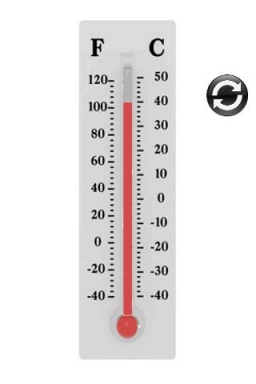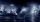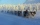# The temperature 6

The temperature was 47°F on Thursday and 60°F on Friday. How much did the temperature rise?

Correct result:

d =  13 °F

#### Solution:We would be pleased if you find an error in the word problem, spelling mistakes, or inaccuracies and send it to us. Thank you!## Next similar math problems:Added together and write as decimal number: LXVII + MLXIV
• SchoolThere are 150 pupils in grade 5 . 2/3 of it are female. By what fractions are the males?
• Flood waterFlood waters in some US village meant that the homes had to evacuate 364 people. 50 of them stayed at elementary schools, 59 them slept with their friends and others went to relatives. How many people have gone to relatives?
• The temperature 2The temperature of Siberia fell13 degrees below 2/3 degrees centigrad. What is the temperature there now?
• Celsius 25 degrees Celsius at midday dropped 12°Celsius degree by evening. What is the temperature?
• Change in temperatureStarting temperature is 21°C, the highest temperature is 32°C. What is the change in temperature?
• The temperature 9The temperature at 9 a. M. is 2 degrees. The temperature rises 3 more degrees by noon. Which expression describes the temperature at noon? Calculate the new temperature value.
• Temperature change 3At 2 p. M. The temperature was 76 degrees Fahrenheit. At 8 p. M. The temperature was 58 degrees Fahrenheit. What was the change in temperature?
• Temperature 13Work out the temperature after each change. The temperature starts at 6°C and falls by 13°C. The temperature starts at 2°C and falls by 8°C
• Storm 3If the temperature yesterday was 56 and today is 13 degrees cooler, what is today's temperature?
• Difference of temperaturesThe temperature on Saturday was –2°C. The temperature on Sunday was 8°C. Write down the difference in these two temperatures.
• The temperature 8The temperature on Monday was –6°C. On Tuesday the temperature was 3 degrees lower. Write down the temperature on Tuesday.
• The temperartureThe temperarture is 0 degrees. It drops 5 degrees after 1 hour and then drops 3 degrees more the next hour. What is the temperature after 6 hours?In about 12 hours in North Dakota the temperature rose from -33 degrees farenheit to 50 degrees farenheit. By how much did the temperature change?The outside temperature changed -14F over 4 hours. If the temperature changed the same amount each hour, what was the change in temperature each hour?The lowest temperature on Thursday was 20°C. The lowest temperature on Saturday was 12°C. What was the difference between the lowest temperatures?Today's temperature was 80 degrees, and then the temperature dropped 10 degrees. Then it dropped 15 degrees again, then the next day, the temperature went up 2 degrees. What would the temperature be?